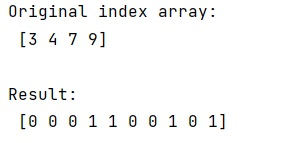# How do I turn an index array into a mask array in NumPy?

Learn, how can we turn an index array into a mask array in Python NumPy? By Pranit Sharma Last updated : March 28, 2023

Suppose that we are given an array of indices that we need to convert into an array of ones and zeros of a given range.

## NumPy - Turning an index array into a mask array

To turn an index array into a mask array, we have a simple trick to create a mask array of 0s and 1s. we will first create an array of zeros of a fixed size and then we will index this array with the array of indices and assign all the values where the index matches as 1.

Also, If the mask is always a range, we can eliminate index_array, and assign 1 to a slice and if we want an array of boolean values instead of integers, we can change the dtype of mask_array when it is created.

Let us understand with the help of an example,

## Python code to turn an index array into a mask array in NumPy

```# Import numpy
import numpy as np

# Creating index array
arr = np.array([3, 4, 7, 9])

# Display Original array
print("Original index array:\n",arr,"\n")

# defining a range
range_ = 10

# Creating zero array

# Display result
```

### Output